# Equations Involving Inverse Trigonometric Functions JEE Notes | EduRev

## JEE : Equations Involving Inverse Trigonometric Functions JEE Notes | EduRev

The document Equations Involving Inverse Trigonometric Functions JEE Notes | EduRev is a part of the JEE Course Mathematics (Maths) Class 12.
All you need of JEE at this link: JEE

Equations Involving Inverse Trigonometric Functions

Ex.1 Solve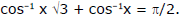Sol.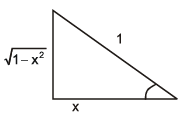Given...(1)

or cos–1 x√3 = π/2 – cos–1 x

or cos cos1 x√3 = cos (π/2 – cos–1x)

or x√3 = sin cos–1x    or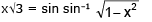or x√3 =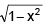Squaring we get 3x2 = 1 – x2  or 4x2 = 1 = x = ± 1/2

Verification : When x = 1/2

L.H.S. of equation = cos1 ( 3 /2) + cos–1 (1/2) = π/6 + π/3 +π/2 = R.H.S. of equation

When x = –1/2

L.H.S. of equation =  cos–1 (– 3 /2) + cos–1 (–1/2) = π – cos–1 ( 3 /2) + π – cos–1 (1/2)

= π – π/6 + π – π/3 = 3p/2 ≠ R.H.S. of equation

∴ x = 1/2 is the only solution

Ex.2 Solve for x : (tan-1 x)2 + (cot-1 x)2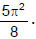Sol.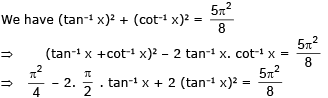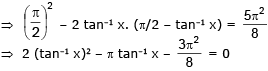tan–1 x = - π/4, 3 π/4  =  tan–1 x = – π/4;  x = –1,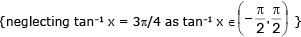Ex.3 Determine the integral values of ' k ' for which the system , (arc tan x)2 + (arc cos y)2π2 k and tan -1 x + cos -1 y = π /2 posses solution and find all the solutions.

Sol.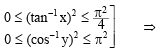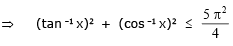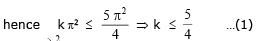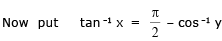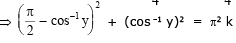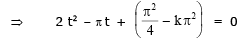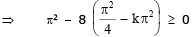= 1 - 2 + 8 k ≥ 0 = k ≥ 1/2 ..(2)

From  (1)  and  (2)    k = 1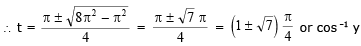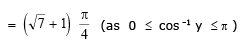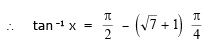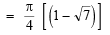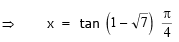G. Inequations involving inverse trigonometric functions

Ex.1 Find the interval in which cos-1 x > sin-1 x.

Sol.

We have, cos–1 x > sin–1 {for cos–1 x to be real; x E [–1, 1]}

2 cos–1 x > π/2  = cos–1 x > π/4   or  cos (cos–1 x) < cos π/4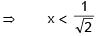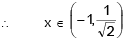Ex.2 Find the solution set of the inequation sin-1(sin 5) > x2 - 4x

Sol.

sin–1(sin 5) > x2 – 4x ⇒ sin–1[sin(5 – 2π)] > x– 4x

⇒ x2 – 4x < 5 – 2π ⇒ x– 4x + (2π – 5) < 0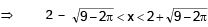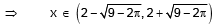Summation of Series

Ex.1 Sum the series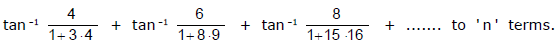Sol.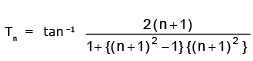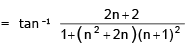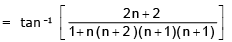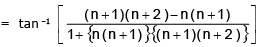= tan -1 (n + 1) (n + 2) - tan -1 n (n + 1)

Put n  =  1 , 2 , 3 , ........ , n and add, we get Sn  = tan -1 (n + 1) (n + 2)  -  tan -1 2

Ex.2 Sum the series to ' n ' terms ,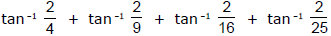+ ...... to ' n ' terms. Also show that , S  = tan -1 3 .

Sol.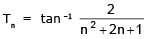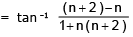= tan -1 (n + 2)  –  tan -1 (n)

Hence, Sn = tan -1 (n + 2)  +  tan -1 (n + 1) - (tan -1 1  +  tan -1 2)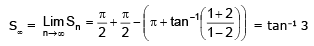Ex.3 If the sum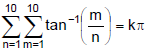, find the value of k.

Sol.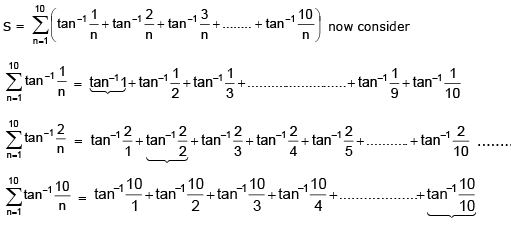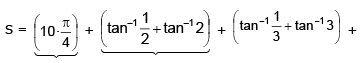.....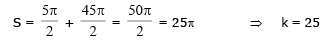Offer running on EduRev: Apply code STAYHOME200 to get INR 200 off on our premium plan EduRev Infinity!

## Mathematics (Maths) Class 12

209 videos|222 docs|124 tests

,

,

,

,

,

,

,

,

,

,

,

,

,

,

,

,

,

,

,

,

,

;